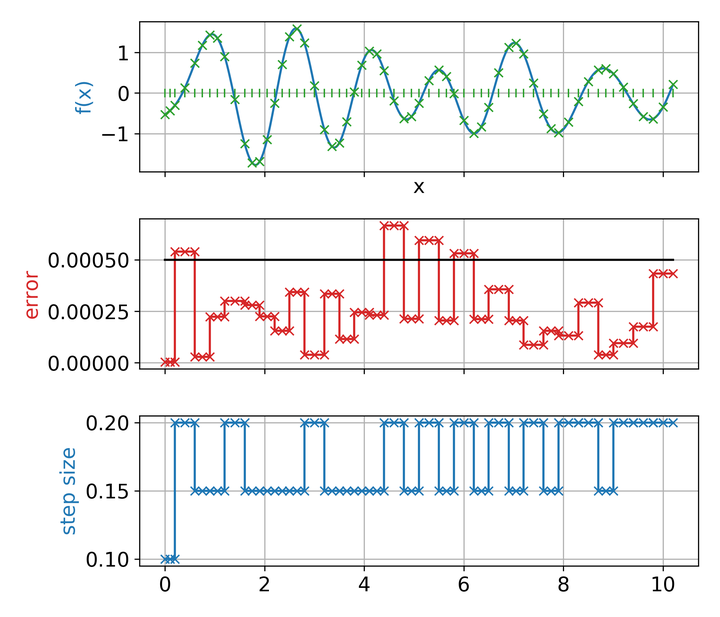# Efficient time stepping for numerical integration using reinforcement learning### Abstract

Many problems in science and engineering require the efficient numerical approximation of integrals, a particularly important application being the numerical solution of initial value problems for differential equations. For complex systems, an equidistant discretization is often inadvisable, as it either results in prohibitively large errors or computational effort. To this end, adaptive schemes have been developed that rely on error estimators based on Taylor series expansions. While these estimators a) rely on strong smoothness assumptions and b) may still result in erroneous steps for complex systems (and thus require step rejection mechanisms), we here propose a data-driven time stepping scheme based on machine learning, and more specifically on reinforcement learning (RL) and meta-learning. First, one or several (in the case of non-smooth or hybrid systems) base learners are trained using RL. Then, a meta-learner is trained which (depending on the system state) selects the base learner that appears to be optimal for the current situation. Several examples including both smooth and non-smooth problems demonstrate the superior performance of our approach over state-of-the-art numerical schemes.

Publication
arXiv.org